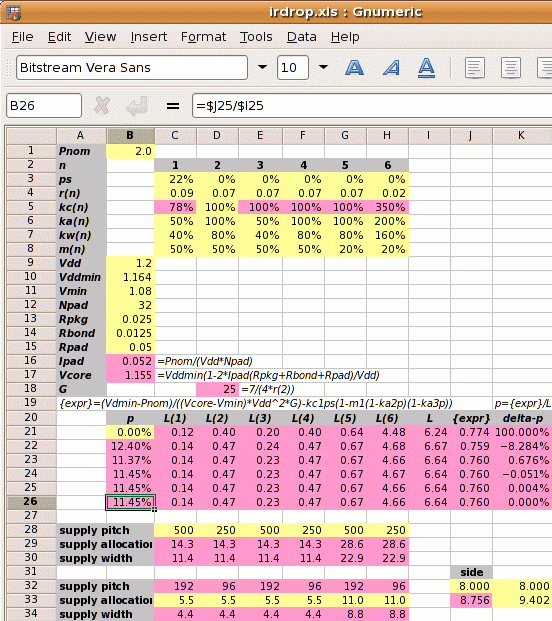The horizontal power straps are half the width (or twice the pitch) of vertical ones; metal resistivities are different; core power consumption is 2W with 32 core Vdd and 32 core Vss pads; 30% core is RAM blocked to metal-4; 20% is analog blocked to all layers.

Step 1: Calculate Ipad and Vcore:

1⁄(1.2×16) = 0.052A
Vcore =
1.164×(1−2×0.052×(0.025+0.0125+0.05)⁄1.2
1.155V

Step 2: Calculate the reference power supply conductance G:

G =
 7 4×r2

7 ⁄ (4 × 0.07) =  25 mhos

Step 3 is to set out the values of kan, kwn, kcn and mn for each metal layer, and use these to calculate the value of L.

metal layer 1 2 3 4 5 6
kan  50% 100%  50% 100%  50% 200%
power metal allocated coefficient
kwn  80%  80%  80%  80%  80%  80%
power metal used coefficient
kcn  78%¹ 100% 100% 100% 100% 350%²
conductivity coefficient
mn  50%  50%  50%  50%  20%  20%
core area blocked
¹78%=.07/.09; ²350%=.07/.02

The value of L depends on p which we don't know. We iterate to the solution and use p=0 for the first estimate.

 L = kw1kc1(1-ps)(1-m1(1-ka2p)(1-ka3p))+ kw2kc2(1-m2(1-ka2p)(1-ka3p))+ kw3kc3(1-m3(1-ka2p)(1-ka3p))+ kw4kc4(1-m4(1-ka2p)(1-ka3p))+ kw5kc5(1-m5(1-ka2p)(1-ka3p))+ kw6kc6(1-m6(1-ka2p)(1-ka3p)) = ( 0.12 + 0.4 + 0.2 + 0.4 + 0.64 + 4.48 ) = 6.24

Step 4: Calculate the power strap allocation percentage p. The solution must be iterated, and the calculation below shows the first iteration.

 m1′ = m1×(1-ka2p)(1-ka3p)
p =
 { Vddmin×Pnom −kc1×ps(1-m1′) } × 1 (Vcore−Vmin)×Vdd2×G L
 { 1.164×2 −0.78×0.22×(1-0.5) } × 1 (1.155−1.08)×1.22×25 6.24
(0.860−0.086)×0.160 = 12.40%

As shown on the right, a spreadsheet can be used to iterate to the answer of p=11.45%.

Step 5: Calculate the new core size. If the initial core size estimate without power straps is x, then with power straps the core size becomes x

 x′ = x = x = x+9.44% √(((1−ka2p)(1−ka3p)) √(0.8855×0.9428)

The value 9.44% is called the IR Drop Adder.

The power strap widths are set by the user defined pitch, strap allocation or width and the value of p just calculated. An example is shown in the table below, where we set the supply strap allocation to 5.5µm and compare it to the old solution.

p width metal pitch core
1-4 5 6 hor ver side
old 19.69% 5.5µm 5.5µm 11µm 112µm  56µm 9.402mm
new  11.45% 5.5µm 11µm 11µm 192µm  96µm 8.756mm

Design Attribute Value
Pnom core power consumption 2W
ps fraction of metal-1 in the standard cells used for power supplies 22% (for vsclib)
r1 resistivity of metal layer 1 in ohms per square 0.09Ω per sq.
r2-5 resistivity of metal layers 2-5 in ohms per square 0.07Ω per sq.
r6 resistivity of metal layer 6 in ohms per square 0.02Ω per sq.
ka1,3,5
 user defined   ratio of metal layers 1,3,5 allocated to power metal-2 allocated to power
50%
ka4
 user defined   ratio of metal layer 4 allocated to power metal-2 allocated to power
100%
ka6
 user defined   ratio of metal layer 6 allocated to power metal-2 allocated to power
200%
m1-4 metal layers 1-4 blocked to power straps 50%
m5-6 metal layers 5-6 blocked to power straps 20%
Vdd the nominal supply voltage 1.2V
Vddmin the minimum supply voltage, 3% less than nominal 1.164V
Vmin the desired voltage at the centre of the die, 10% less than the nominal 1.08V
Rpkg the resistance of the package leadframe 25mΩ
Rbond the resistance of the bond wire 12.5mΩ¹
¹double bond halves bond wire resistance; ²two supply pads halves pad resistance

kcn =
 r2 rnThe new solution has allowed the vertical power strap pitch to go up from one every 56µm to one every 96µm. The core side is 646µm less and the core area is 13% less than the first solution, due to double bonding of supply pads, tighter Vddmin spec and wider metal-5 straps.